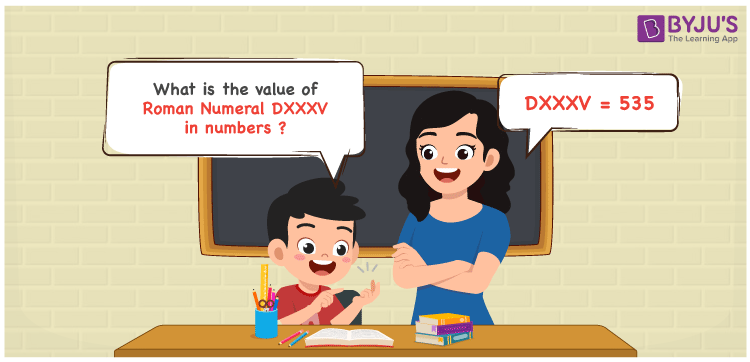Checkout JEE MAINS 2022 Question Paper Analysis : Checkout JEE MAINS 2022 Question Paper Analysis :

# DXXXV Roman Numerals

DXXXV Roman Numerals is 535. Roman Numerals are the process of representing numbers using the Roman alphabet. Students, who aspire to speed up their problem-solving skills and score optimum marks in exams are advised to practise problems using the PDF of Roman Numerals 1 to 50 on a daily basis. Learn the conversion of DXXXV Roman Numerals into numbers in a detailed manner in this article.

 Number Roman Numeral 535 DXXXV

## How to Write DXXXV Roman Numerals?We can express the numerical value of DXXXV Roman Numerals by following any of the two methods given below

First Method:

• Break the Roman Numerals into single letters
• DXXXV = D + X + X + X + V
• Write the numerical value of each letter and add/subtract them
• DXXXV = 500 + 10 + 10 + 10 + 5 = 535

Second Method:

• In this method, we consider the groups of Roman Numerals for addition or subtraction
• DXXXV = D + XXX + V = 500 + 30 + 5 = 535
• Therefore the numerical value of DXXXV Roman Numerals is 535

## Video Lesson on Roman Numerals## Related Articles

Roman Numerals

Roman Numerals 1 to 100

Roman Numerals 1 to 1000

Roman Numerals Conversion

L Roman Numeral

Roman Numeral XXXIX

## Frequently Asked Questions on DXXXV Roman Numerals

### How do you write DXXXV Roman Numerals in numbers?

DXXXV Roman Numerals in numbers is written as 535.

### Find the product of DXXXV and II Roman Numerals.

DXXXV = 535 and II = 2. 535 x 2 = 1070. Since 1070 = MLXX. Hence the product of DXXXV and II Roman Numerals is MLXX.

### What is the value of (100 – 85) + 535 in Roman Numerals?

(100 – 85) + 535 = 550. The number 550 in Roman Numerals is DL. Therefore the value of (100 – 85) + 535 in Roman Numerals is DL.